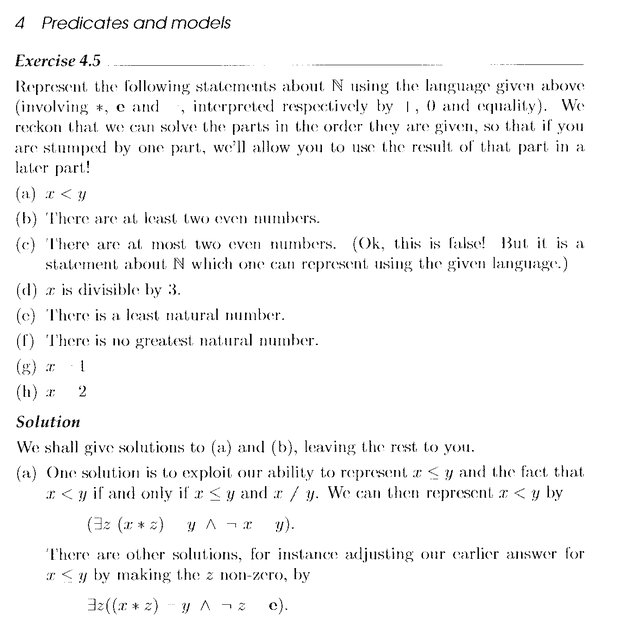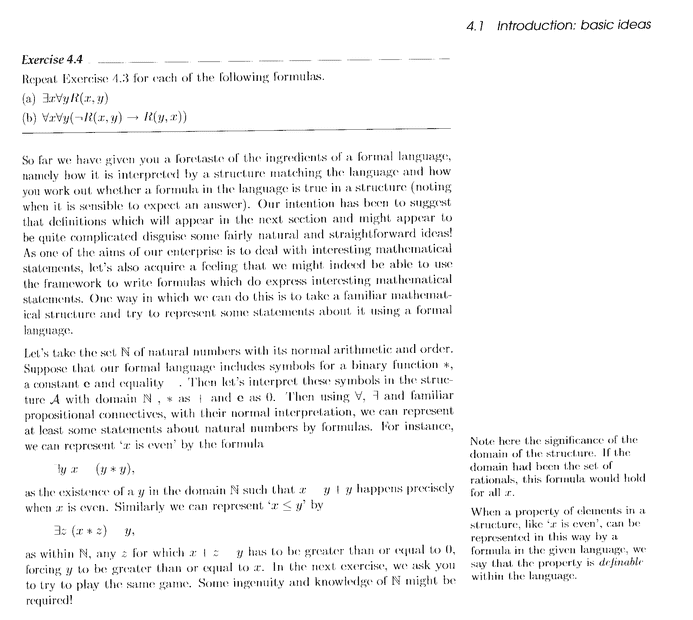# Predicates and Models ... Goldrei

Gold Member
MHB
I am reading Goldrei's book: "Propositional and Predicate Calculus: A Model of Argument", Chapter 4: Predicates and Models.

I need help in understanding Goldrei's answer to Exercise 4.5 (a) ...

Exercise 4.5 together with Goldrei's solution reads as follows:Can someone please explain exactly how x < y can be represented by

## ( \exists z \ (x * z) = y \land \neg x = y ) ##

Help will be much appreciated.

Goldrei mentions the language given above ... that reads as follows:Peter

Mentor
Your scanned or photographed images are difficult to read, especially because some symbols don't show up.
In the image for ex. 4.5, it says "(involving *, e and , interpreted respectively by 1 (?), 0, and equality)." It looks like whatever was written after "and" doesn't show up. Possibly it was in a different color. Also, is the first symbol after "respectively by" the digit 1 or the symbol |? It looks like the latter, but I don't know what that means in this context.

Can someone please explain exactly how x < y can be represented by
##(\exists z (x * z) = y \wedge \neg x = y)##
The text in the second image is smaller, so more difficult to read. Several symbols in the explanation in this image also don't show up, so I still don't understand what is meant by *.

Gold Member
* represents addition (I agree it's very tough to read). In more standard mathematical language:
$$( \exists z \ (x * z) = y \land \neg x = y )$$
says that there's a ##z## such that ##x+z=y## and ##x\neq y##. Alternatively, the other explanation
$$( \exists z \ (x * z) = y \land \neg z = \mathbf{e} )$$
says that there's a ##z## such that ##x+z=y## and ##z\neq 0##.

•Math Amateur
SSequence
Alternatively, the other explanation
$$( \exists z \ (x * z) = y \land \neg z = \mathbf{e} )$$
says that there's a ##z## such that ##x+z=y## and ##z\neq 0##.
For clarity of notation, I think it is probably better to have the ##z## variable to be outside all of the brackets.

===========

One thing to note about these formulas is that since the predicate is of the form ##less(x,y)##, any variables other than ##x## and ##y## that are used must be bound. This is true in general regardless of the specific predicate so it is worth a mention [since checking for it can sometimes help identify a mistake in more complicated formulas].

Also, I agree that it is not difficult to understand the solution if you write it with more familiar symbols.
(1) ##\exists z [x+z=y] \land (x\neq y)##
(2) ##\exists z [(x+z=y) \land (z\neq 0)]##

In the first formula if you look separately at:
##\exists z [x+z=y] ##
then this is just the representation of predicate ##x \leq y##. So the first formula is just saying that ##x<y## iff we have (i) ##x \leq y## and (ii) ##x \neq y##.

Last edited:
•Math Amateur
Gold Member
For clarity of notation, I think it is probably better to have the z variable to be outside all of the brackets.
I agree, but that’s how it was written in the book. Not sure why.

SSequence
I agree, but that’s how it was written in the book. Not sure why.
Given the first image posted in OP, the book does put ##\exists z## outside all of the brackets in the second formula!

Anyway, it also occurred to me that formula-(1) in post#4 also works [as in correctly describes "less than" predicate] even if the domain of quantification is ##\mathbb{R}## or ##\mathbb{Q}##. However, while formula-(2) works for ##\mathbb{N}##, it doesn't work for ##\mathbb{R}## or ##\mathbb{Q}##.

•TeethWhitener and Math Amateur
SSequence
Anyway, it also occurred to me that formula-(1) in post#4 also works [as in correctly describes "less than" predicate] even if the domain of quantification is ##\mathbb{R}## or ##\mathbb{Q}##.
Sorry for the mistake. Formula-(1) in post#4 doesn't represent the "less than" predicate either [if the domain of quantification is ##\mathbb{R}## or ##\mathbb{Q}##]. It represents the "not equal" predicate in that case.

In fact, if we look at it:
(1) ##\exists z [x+z=y] \land (x\neq y)##
Then basically it is just equivalent to the predicate ##x\neq y## if the domain of quantification is ##\mathbb{R}##, ##\mathbb{Q}## or ##\mathbb{Z}##.

And while we are at it, we might also look at the second formula:
(2) ##\exists z [(x+z=y) \land (z\neq 0)]##
This formula is also equivalent to the predicate ##x\neq y## if the domain of quantification is ##\mathbb{R}##, ##\mathbb{Q}## or ##\mathbb{Z}##.

Last edited: